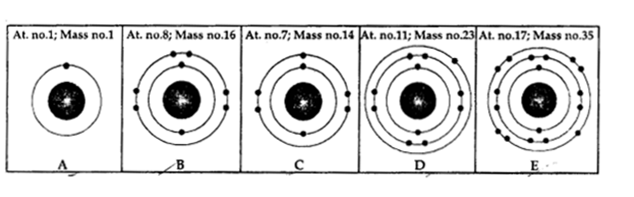# New Simplified Chemistry Class 9 ICSE Solutions Atomic Structure

## New Simplified Chemistry Class 9 ICSE Solutions Atomic Structure

Viraf J Dalal Chemistry Class 9 Solutions and Answers

Exercise

Question 1.(1984)
Define the terms : atomic number, mass number and electron.
Atomic number [Z] : “Is the number of protons in the nucleus of an atom.”
OR “Is the number of positive charges in the nucleus of its atom.”
OR “Is the number of electrons in the complete atom.”
Z = P = e
Mass number [A] : “Is the total number of protons and neutrons in the atom of an element.”
A = P + n
Electron [e] : “A sub-atomic particle having a unit negative charge and mass equal to 1/1837 of that of hydrogen atom, revolve round the nucleus.” [-1e0]

Question 1.(1985)
From the symbol$\begin{matrix} 4 \\ 2 \end{matrix}$ He for the element helium, write down the mass number and the atomic number of the element.• Mass number — 4
• Atomic number — 2

Question 1.(1986)
Sulphur has an atomic number of 16 and a mass number of 32. State the number of protons and neutrons present in the nucleus of sulphur.
Protons → Atomic number = 16 = P
Number of Neutrons = A – P = 32 – 16 = 16

Question 2.(1986)
Give a simple diagram to show the arrangement of the electrons in an atom of sulphur.
In sulphur atom.
Number of electrons = P = e = 16Question 1.(1987)
2412Mg and 2612Mg are symbols of two isotopes of magnesium. Compare the ‘ atoms of these isotopes with respect to :

1. the composition of their nuclei.
2. their electronic configurations.
3. give the reason why the two isotopes of magnesium have different mass numbers.Question 2.(1987)
Chlorine is an element of atomic number 17. It is a mixture of two isotopes having mass number of 35 and 37.

1. What is meant by “atomic number of an element” ? What do you understand by an ‘atom’
2. Write down the electronic configuration of the chlorine atom.
3. State the number of protons, electrons and neutrons in the following isotopes: 35Cl17, 37Cl17
4. Explain why the two atoms in (iii) above have the same chemical reactions.
5. If molten magnesium chloride is electrolysed suggest a suitable electrode [anode].

1. Atomic number is the number of protons in an atom.
Atom : The basic unit of matter is the smallest particle of an element which can take part in a chemical reaction.
2. Number electrons in chlorine atom is 17 [2, 8, 7] and their distribution in various shells in K = 2, L = 8, M = 7.
3. Two isotopes of chlorine are
3517Cl number of protons = 17 and number neutrons = 35 – 17 = 18
Number of electrons = 17
3717 Cl number of protons = 17
electrons = 17
neutrons = 20 (37 – 17)
4. Two isotopes of chlorine have same chemical reactions as their atomic number i. e. number of electrons i.e. electronic configuration is same (17).
5. When molten magnesium chloride is electrolysed suitable anode used is carbon.

Question 1.(1988)
Five atoms are labelled V to Z1. Which one of these atoms (1) contains 7 protons ; (2) has an electronic configuration 2, 7 ?
2. Write down the formula of the compound formed by atoms X and Y.

(i)
1.
Contains 7 protons
Z – has atomic number of Z = 7
2. Has atomic configuration (2, 7)
W – atomic number = of electrons = 9 (2, 7)
(ii) X-atomic number 3
= number of electron = (2, 1)
∴ X has valency [1+]
Y-atomic number = 8
= number of electrons = (2, 6)Question 1.(1989)
Elements X, Y, Z have atomic numbers 6, 9 and 12 respectively. Which one :

1. forms anion – negative ion ;
2. forms cation – positive ion ;
3. has 4 electrons in the outermost orbit. [Y, Z, X]

Atomic number of
X = 6 = number of electrons (2, 4)
∴ X has 4 electrons in outermost orbit.
Y = 9 = number of electrons (2, 7)
∴ Y form anion = negative ion
Z = 12 = [2, 8, 2]
∴ Z forms cation – positive ion.

Question 2.(1989)
Ordinary chlorine gas has two isotopes : 3517Cl and 3717Cl in the ratio of 3 : 1. Calculate the relative atomic mass [atomic weight] of chlorine.Question 1.(1990)
The atom of aluminium is represented by 27Al13. Write down the number of

(a) electrons
(b) protons
(c) neutrons
(d) the arrangement of electrons

in the different orbits or shells in one atom of aluminium.Question 2.(1990)
Name the clement which does not contain any neutrons in its nucleus.
Element is [H] Hydrogen.

Question 3.(1990)
Elements A, B and C have atomic numbers 9, 20 and 10 respectively.

1. State which one is (1) a non-metal, (2) a metal, (3) chemically inert.[A,B,C]
2. Write down the formula of the compound formed by two of the above elements.[BA2]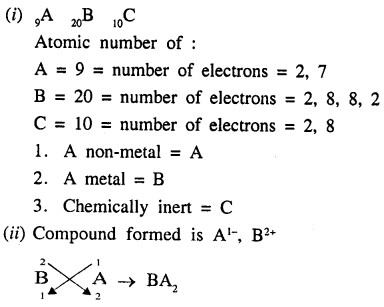Question 4.(1990)
Define : Proton, Electron, Neutron.
Proton is subatomic particle found in nucleus of atom having unit positive charge, mass = 1 hydrogen atom.
Found in nucleus of atom and number of protons in an atom = number of electrons.
Electron = e = is sub atomic particle revolving around the nucleus of atom having
unit – ve charge, mass = 1/1840 of 1 hydrogen atom and number of electrons = number of protons.
Neutrons = n = is sub-atomic particle found in nucleus of atom, having no charge, mass = mass of 1 proton.

Question 1.(1991)
Write down the electronic configuration of the following (i) 2713X, (ii) 3517Y.
Write down the number of electrons in X and neutrons in Y and the formula of the compound formed by X and Y.[XY3]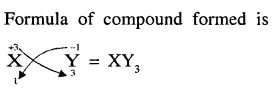Question 1.(1992)
According to the Dalton’s Atomic Theory, atoms of the same element are identical in all respects. But according to the Modern Atomic Theory, this postulate is proved wrong. Explain.
According to modern atomic theory atoms of the same element may not be alike in all respect.
e.g. isotopes of chlorine 3517Cl and 3717 Cl have different atomic masses 35 and 37. This proves Dalton’s Atomic Theory wrong.

Question 1.(1993)
What are isotopes.
Isotopes : “Atoms of the same element having same atomic number, different mass number and similar chemical properties.”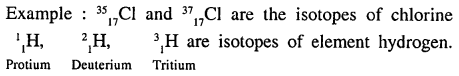Question 2.(1993)
Write down :

1. the mass number of the atom having 20 neutrons and 15 protons.
2. the number of neutrons in the nucleus of an atom having atomic number 17 and mass number 37.

1. Mass number (A) = Neutrons (n) + Protons (p)
Mass number = 20 + 15 = 35
2. A = n + p (At. No.) A is mass number
37 = n + 17
Number of neutrons = n = 37 – 17 = 20

Question 1.(1994)
What is a proton ? What is the significance of the number of protons found in the atoms of each of the different elements.
Proton : “Is positively charged sub-particle of atom present in nucleus.”
Proton helps in determining and understanding the structure and behaviour of an atom. Elements are arranged on the bases of number of protons in their respective atoms.

Question 2.(1994)
What is the relation between the number of protons and the number of electrons in an atom.
The number of protons in an atom is equal to the number of electrons.

Question 3.(1994)
What would be the reason for an element to have atoms with differing mass numbers.
Atoms of same element have different mass number as these isotopes have different physical constants i.e. different physical properties. OR “Atoms have different number of neutrons.”

Question 4.(1994)
Copy and complete the following table relating to the atomic structure of some elements :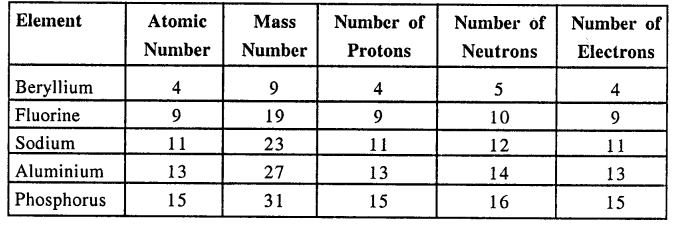Question 5.(1994)
The electronic structure [configuration] of fluorine can be written as 2, 7. In a similar way give the electronic configuration of :

1. aluminium
2. phosphorus

Electronic configuration of :

1. Aluminium 13 is 2, 8, 3.
2. Phosphorus 15 is 2, 8, 5

Question 1.
State the main postulates of Dalton’s atomic theory. How does the modern atomic theory contradict and correlate with Dalton’s atomic theory.
Postulates of Dalton’s Atomic Theory :

1. Matter consist of small indivisible particles called atoms.
2. Atoms are neither created nor destroyed.
3. Atoms of the same element are alike in every respect i.e. atoms of oxygen are similar (have same At. No. Mass No. etc.) and atoms of hydrogen are similar.
4. Atoms of different elements are different in every respect i.e. atoms of hydrogen atoms are different from atoms of oxygen.
5. Atoms combine with other atoms in simple whole number ratio forming compound atom or molecules.

1. Atoms are no longer indivisible. Atoms are made up of subatomic particles protons, electrons and neutrons.
2. Atoms can be changed in energy.
3. Atoms of same elements may not be alike i.e. isotopes 126C 136 C 46C.
4. Atoms of different elements may be similar i.e. isobars.
5. Ratio in which atoms combine may not be simple whole number ratio i.e. ratio of atoms in C12 H22 O11 (sugar) is not simple whole number.

Question 2.
Explain in brief the experimental proofs which led to the discovery of –

1. Electrons
2. Protons
3. atomic nucleus
4. neutrons.

(i) Electrons : English physicist William Crookes in 1878 discovered the cathode raysHe found that when an electric discharge is passed through a tube containing a gas at low pressure (0.01 mm of Hg).
Blue rays were emitted from the negative plate [i.e. cathode]. These rays were called cathode rays and consist of negatively charged particles now called electrons. Electrons are essential part of each and every atom.
(ii) Protons : Since atoms are electrically neutral there must be equal positively charged particles in atom. This led to discovery of the protons.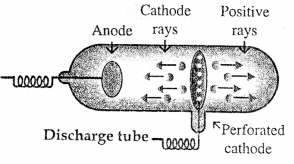Goldstein discovered protons. He used a perforated disc as cathode and found positively charged rays travelling in opposite direction to cathode rays.
These were protons i.e. positively charged particles, 1837 times heavier than an electron.
(iii) Discovery of Atomic Nucleus : Lord Rutherford in 1911 directed alpha particles (42He) towards gold foil. The deflection of the alpha particles was observed and concluded that there was nucleus at the centre of atom which was positively charged dense very small space (solid) called nucleus.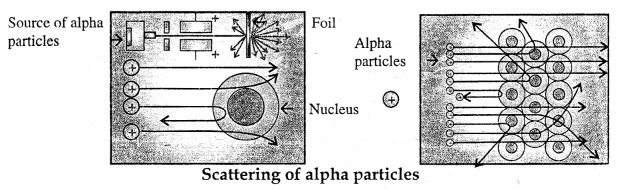(iv) Discovery of Neutrons : As atom contains protons and electrons. Atomic mass of electron in negligible.
∴ An atom of helium which contains 2 protons should have a mass = (2 × 1) + 0 = 2 a.m.u. But the atomic mass of a helium atom was found to be approximately 4.0 a.m.u. It was therefore proved that, in the nucleus of an atom, there is an other particle called a neutron which has no electrical charge but is almost of an equal mass as the protons.

Question 3.
State in brief the drawbacks of Rutherfords atomic model correlating them with the postulates of Bohr’s atomic model.
Drawbacks of Rutherfords’s Atomic Model :

1. The comparison of electrons with the planets in the solar system is the main drawback of Rutherford’s model. Because electrically charged particles in motion lose energy (radiate energy). As a result electrons revolving the nucleus should after losing energy, fall in spiral path and colLapse.
2. If it was so atom should be highly unstable, but we know that atom is structurally stable. Rutherford’s model could not explain this stability.Postulates of Bohr’s Atomic Model :

1. Electrons revolve around the nucleus in fixed orbits or energy levels or shells.
2. While revolving around the nucleus in an orbit, an electron does not lose energy nor does it gain energy.
3. The integer ‘n’ represents the various energy levels 1, 2, 3 or k, l, m starting from the innermost.
4. An electron revolving in a particular orbit, on gaining a certain amount of energy, jumps to the next orbit and vice versa.
These postulates explain the cause of stability of the atom.

Question 4.
What is meant by the terms :

1. subatomic particles,
2. nucleus,
3. orbits,
4. atomic number
5. mass number with reference to an atom.

1. Subatomic particles : “Particles into which atom is divided are called subatomic particles.” Protons, electrons, neutrons.
2. Nucleus : “Subatomic part of atom which is very small as compared to the size of, atom +vely charged, massive and dense present at the centre of atom.”
3. Orbits : “Shells into which electrons revolve around the nucleus.”
4. Atomic number : “Number of protons or numbers of electrons present in an atom.” [Z]
5. Mass number [A] : “Total number of protons + number of neutrons” A = p + n.Question 5.
Represent each of the following :

1. a proton ‘p’
2. an electron ‘e’
3. a neutron ‘n’ in terms of its symbols showing the subscript and superscript values.Question 6.
What arc ‘energy levels’ ? Explain the arrangement and distribution of electrons in the various shells with reference to an atom in general and to an atom of potassium ‘3919K’ with special referencence to the 2n2 rule.
Energy levels : ‘Electrons revolved the nucleus in definite orbits called shells or energy levels since while revolving in a shell an electron possesses a certain amount of energy.”
Arrangement of electrons : Direction of electrons in various shells is given by a formula 2n2 where is the number
n = 1 In first shell there can be maximum of 2 × (1)2 = 2 electrons.
n= 2 In second shell there can be maximum of 2 (2)2 = 8 electrons.
n = 3 In third shell there can he maximum of 2 (3)2 = 2 × 9 = 18 electrons and soon.
But there cannot be more than 8 electrons in the outermost shell and cannot be more than 18 electrons in penultimate orbit
Higher shell will start only when the lower shells are completely filled.
3919k has 19 electrons
In first shell or K shell will have 2 electrons.
2nd shell or L shell 8 electrons.
3rd shell or M shell 8 electrons
and 4th or N shell 1 electron.

Question 7.
An element ‘A’ has mass number 23 and atomic number 11. State the —

1. no. of neutrons in its shell,
2. electronic configuration of the element ‘A’.

Mass number A = n + p (atomic number)
A = n + Z
23 = n + 11
∴ n = 23 – 11 = 12
(i) Number of neutrons = 12
(ii) e = p = 11
∴ Number of electrons e = 11
∴ Electron configuration of A = 2, 8, 1

Question 8.
The following elements U to Z are given 3U, 6V, 9W, 14X, 18Y, 20Z.
State the electronic configuration of each and state whether they arc metals, non-metals or inert gases.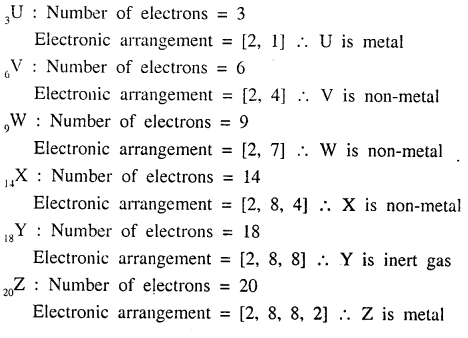Question 9.
Draw the geometric atomic structure of each of the following atoms showing the number of electrons, protons and neutrons in each of them :

1. 126 C
2. 2311 Na
3. 3115 P
4. 3919 K
5. 4020 Ca.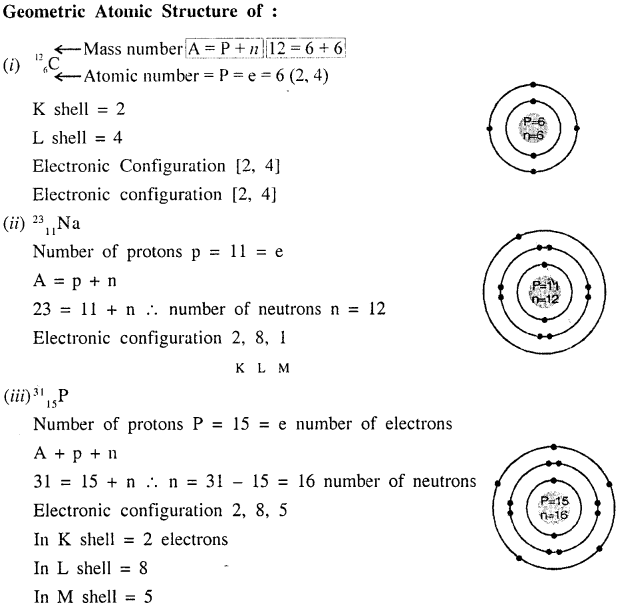Question 10.
Define an ‘isotope’. Give reasons why isotopes have same chemical but different physical properties.
Isotopes : “Are the atoms of the same element having same atomic number but different mass number.”
Chemical properties are same because they have same atomic number hence same number of valence electrons physical properties are different because they have different mass number.

Question 11.
Draw the geometric atomic structure of the three isotopes of hydrogen and the two isotopes of chlorine.
Geometric atomic structures of three isotopes of hydrogen.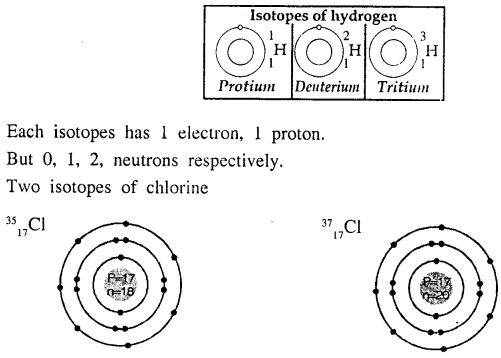Question 12.
Four elements A, B, C, D are given :
A shows the presence of 20 neutrons, 17 protons and 17 electrons.
B shows the presence of 18 neutrons, 17 protons and 17 electrons.
C shows the presence of 10 neutrons, 9 protons and 10 electrons.
D shows the presence of 4 neutrons, 3 protons and 2 electrons.
State which of the above is —

(a) an anion
(b) a cation
(c) a pair of isotopes.

Write the formula of the compound formed between D and C.
For (a) and (b) and (c) we need to find the valence electrons.
Element A has 17 electrons [2, 8, 7]
As there are 7 electrons in outermost shell. It will gain 1 electron to attain stable octet structure of nearest gas — Neon [2, 8] ∴ A1- is Anion.
Element B also has 17 electrons [2, 8, 7] is short of 1 electron in the valence shell will gain 1 electron and B is Anion.
Element C has 10 electrons and electronic configuration [2, 8] has 8 electrons in valence shell. Its octet is complete, but there are 9 protons.
∴ Element C must have 9 electrons
The 10th electron is gained to have stable octet structure and hence 8 electrons in valence shell.
∴ C is Anion.
Element D has protons 3 and electrons 2
It should have 3 electrons. This means it has lost 1 electron from valence shell to have a stable duplet structure of nearest gas (inert)  Helium.
∴ D+ is Cation.
(c) A and B are isotopes as both have same atomic number but different number of neutrons.
(d) D1+ C1-
∴ Compound formed is [DC]

Question 13.
What are noble gases. Give a reason why noble gases have stable electronic configuration.
Noble gases are gases which have their outermost orbit completely filled i.e. have stable electronic configuration. They do not gain or lose or share electrons and noble gases have stable electronic configuration as they are in minimum state of energy.

Question 14.
Explain the reason for chemical activity of an atom with reference to its electronic configuration.
Reason for chemical activity of atom is its unstable electronic configuration.

Question 15.
Differentiate between the terms —

(a) Stable and unstable electronic configuration
(b) Duplet and octet rule.

(a) Stable electronic configuration : Atoms having octet (8 electrons) or duplet 2 electrons in outermost shell is stable electronic configuration 2 electrons is K shell the valence shell.
i.e. “Atoms having valence shell completely filled” shell.

• Unstable electronic configuration : “Atoms of element which do not have their valence shells completely filled.”

(b) Duplet Rule : For an atom to achieve stable electronic configuration it must have 2 electrons in the first shell [outermost if it is K shell] like nearest noble gas Helium.

• Octet Rule : For an atom to achieve stable electronic configuration it must have 8 electrons in outermost orbit like that of noble gas other than Helium.

Question 16.
Explain the octet rule for formation of —

(a) Sodium chloride from a sodium atom and a chlorine atom.
(b) Nitrogen molecule from two nitrogen atoms.

(a) Formation of sodium chloride NaCl, Na atom has atomic number 11 [2, 8, 1], There is 1 electron in outermost M shell it is not possible to gain 7 electrons and to lose 1 electron is easier, by losing sodium atom because Na+ ion.
While Cl atomic number 17 [2, 8, 7] has 7 electrons in outermost M shell for Cl atom it is easier to gain 1 electron, becomes Cl ion.
By losing 1 electron and by gaining 1 electron both sodium and chlorine attain octet configuration and become stable and there is electrostatic force which pulls them and become sodium chloride.Each atom of nitrogen has 5 electrons in outermost orbit. For both it is not possible to gain 3 electrons or lose 5 electrons to attain nearest inert gas electronic configuration. So they share 3 electrons each and attain octet configuration which is stable and form N2 molecule.

Atomic Structure – Unit Test Paper 4

Q.1. Select the correct answer from the answers in brackets to complete each sentence.

Question 1.
An element has electronic configuration 2, 8, 1 and 12 neutrons. Its mass no. is ____ [11 / 23/ 12]
An element has electronic configuration 2, 8, 1 and 12 neutrons. Its mass no. is 23.

Question 2.
The maximum number of electrons in M-shell is ____ [8 / 32 / 18]
The maximum number of electrons in M-shell is 18.

Question 3.
Isotopes have same ____ [no. of neutrons / electronic configuration / atomic masses].
Isotopes have same electronic configuration.

Question 4.
An ____ [atom / ion] is capable of independent existence in solution.
An ion is capable of independent existence in solution.

Question 5.
An atom with electronic configuration 2, 7 and mass number 19 will have ____ neutrons. [8 / 10 / 12]
An atom with electronic configuration 2, 7 and mass number 19 will have 10 neutrons.

Q.2. Give reasons for the following.

1. The physical properties of isotopes of the same element are not identical.
2. The mass number of an atom is slightly less than the actual atomic mass.
3. The shells surrounding the nucleus of an atom are also called ‘energy levels’.
4. Helium is chemical extremely unreactivc.
5. Mass number is slightly less than the actual atomic mass.

1. Physical properties depend on mass number and isotopes have different mass numbers.
2. Atomic mass = number of protons + number of neutrons + number electrons, and mass number = protons + neutrons
∴ Atomic mass = mass number + mass of electrons
Though mass of electrons is very small 1/1837 of hydrogen atom, yet the mass of electrons is there.
∴ Mass number is slightly less than atomic mass.
3. Shells are called energy levels since while revolving in a shell an electron possesses a certain amount of energy.
4. Helium has its outermost shell completely filled i.e. 2 electrons in K shell.
5. Same as answer (2) of this question above.

Q.3. Differentiate between the following terms.

1. Electron and proton
2. Atomic number and mass number
3. Nucleus and nucleons
4. Valence shell and penultimate shell
5. Octet and duplet.

Differentiate between :
(i) Electron :

1. e has unit -ve charge
2. has 1/1837 th of 1 hydrogen atom mass negligible.
3. Revolve around nucleus in different shells.

Proton :

1. 11 P has unit +ve charge
2. has unit mass i.e. mass equal to 1 hydrogen atom
3. Are present in nucleus.

(ii) Atomic number :

1. Z-is the number of protons or number of electrons in an atom.
2. Present in nucleus = P
Present in orbits = e

Mass number :

1. A is the sum of protons and neutrons.
2. Present in nucleus of atom.

(iii) Nucleus :

1. Nucleus is central space of atom. Is solid massive, positively charged, dense and very-very small as compared to size of atom.

Nucleons :

1. Is the sum of protons and neutrons, present in nucleus.

(iv) Valence shell :

1. Is the outermost shell containing free electrons.

Penutimate shell :

1. Is the shell before the valence shell.

(v) Duplet :

1. Having 2 electrons in the outermost shell -K shell (if outermost) is called duplet i.e. Helium.

Octet :

1. Having 8 electrons in the outermost shell except He is called octet i.e. Ne, Ar, Kr, Xe, Rn.

Q.4. Name or state the following.

1. The three isotopes of hydrogen.
2. Two elements having same number of protons and electrons but different number of neutrons.
3. The valency of an element whose electronic configuration is 2, 8, 3.
4. The shell closest to the nucleus of an atom.
5. An element having valency ‘zero’.Q.5. State the number of neutrons in each of the atoms A to E. Also state which of the atoms A to E is a metal.